# Mastering Linear Equations and Inequalities | Cambridge IGCSE Mathematics[Please watch the video attached at the end of this blog for a visual explanation on Mastering Linear Equations and Inequalities]

Being yet another subtopic under the overarching branch of algebra, Linear Equations and Inequalities are two areas that are tested each year in every single paper.

### What is an Inequality?

An inequality is any expression in Mathematics that compares two values, whether one value is greater than the other, smaller than the other, not equal to the other, etc. We can use symbols to represent these.If you look at the picture above and consider the first example of x > 5, this means that x is greater than 5, and therefore x can be any number that is greater than 5.

The next example of y < 3 shows that all the values for y will be smaller than 3.

In the third example of x ≥ 8, this means x will have a value of 8 or any value greater than 8.

The last example of y ≤ 10, this means y will have a value of either 10 or a number less than 10.

### How do we solve inequalities?

Inequalities and linear equations are solved in very much the same way.
1. Bring all the s to one side.
2. Bring the numerical terms to the other side.
3. Solve it the way you would a normal equation.

###### Example 1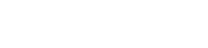In the given question, we need to move – 2x to the right hand side first, so that we can make it + 2x, and then we need to move the 2 to the left hand side to make it – 2. This leaves the values on the right hand side to be 2x and the values on the left hand side to be 2. When solving it the way one would solve a normal equation, we divide both sides by 2, leaving us with x = 1.###### Example 2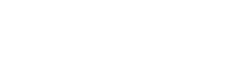In this question, before moving any numbers around, we first need to expand the expression on the left hand side. This expands as 2x + 2, and then this can be moved so that the x terms are on the left hand side and the numbers are on the right hand side. This leaves us with 2x – x > -7 – 2. This means that x > -9, meaning that x is greater than negative 9.### Number Lines and Inequalities

Inequality expressions can always be denoted basically inequality expressions on a number line.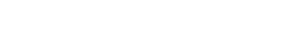We use the shaded circle when the number is included (8 or more than 8), and the empty circle when the number is not included (greater than 8).

###### Example: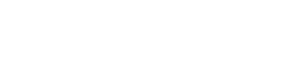The first example tells us that x is less than 4, therefore, since the inequality does not include the number 4, we draw an empty circle on number 4 on the number line, and draw an arrow towards the left hand side (numbers that are less than 4).

The next example shows us an inequality where the number given is included. This inequality reads that x is greater than or equal to -2. Therefore here, we draw a solid/shaded circle on -2 on the number line, and then draw an arrow towards the right hand side (numbers that are greater than -2).

There are also instances where x can take values between a certain range. This requires a bit more attention to be paid to the number line, therefore be sure to watch the video below to learn how to solve inequalities like these!

### Inequalities and Graphs

Inequalities can be represented even in a graph.The above example inequality y > x + 5 can be treated as y = x + 5, which then looks like a y = mx + c equation. In this case therefore, if we consider the y = x + 5 equation, we know that this is a line intersecting at 5 on the y axis, at the y intercept. It is also a positively sloping graph.

This brings us to the question of how to shade an expression with an inequality. Once the y intercept is figured out (+5 in this case), the next thing we must look at is the symbol and whether it is including (≤ or ≥) or not including (< or >). If it does not have including symbols, then we must use a solid line when drawing the graph. If it does not have including symbols, then we must use a dotted line to draw the graph. Then looking at the symbol, if it tells us the value is greater than the number, then we shade above the line, if it is less than the number, then we shade below the line.

### Solving Linear Equations

A linear equation is an equation in which the power of the variable is 1. Equations which start with x2 or y2 are not linear equations.

Solving a linear equation is quite similar to solving an inequality.
1. Move any negative x terms to the right side or left hand side so that they become positive.
2. Move all the numerical values to the opposite side of the x terms.
3. Simplify the equation.

● If there is a fraction, then the final answer can be left as a fraction, albeit simplified into its simplest form.### Linear Equations and Word Problems

Another area that we need to be aware of is on how to come up with simple linear equations to solve word problems at exams.

###### Example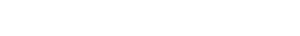Here, we can try and come up with a particular expression to represent the odd numbers. When thinking about odd numbers, the simplest ones are 1,3 and 5. Therefore, if we take x as the first odd number, it can be seen that the second odd number is x +2 (3), and the third odd number is x + 4 (5), and that will be the same no matter which consecutive odd number set is taken. Even if we consider 5, 7 and 9 as the three consecutive odd numbers, this particular formula works for that as well: If x is 5, then x + 2 = 7, and x + 4 = 9. Therefore, we can stick to these variables to figure out the answers.

Therefore, according to the question given above, x + (x + 2) + (x + 4) = 105. This can be simplified and solved as shown below and we can obtain the three consecutive odd numbers to be 33, 35 and 37!### Mastering Linear Equations and Inequalities!

Linear Equations and Inequalities can be confusing to first time students. However, once you practise as many questions as possible, this will be quite simple. Some questions can be found here as well, and you can time your answers to see if you can stick to the time limit given.

If at all you are not sure about whether the line that needs to be drawn is a dotted line or a solid line, or whether you should shade upwards or downwards, etc, it is always advisable to use a pencil and not a pen 🙂

If you are struggling with IGCSE revision or the Mathematics subject in particular, you can reach out to us at Tutopiya to join revision sessions or find yourself the right tutor for you.

Watch the video below for a visual explanation of the lesson on mastering linear equations and inequalities. Don’t forget that there is a timed quiz to test whether you have understood the lesson!